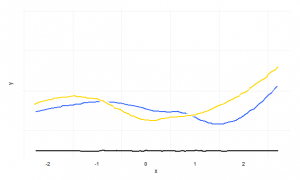Categories

Richard Feynman’s letter to his student

No problem is too small or too trivial if we can really do something about it.

For the full text:

Categories

Array construction in R, Perl, and Python

Array construction in R, Perl, and Python

R:

data

Perl:

@data = (1, 2, 3)
@data[2..3]
%hash = ('a' =>; 'b')
Categories

Debugging R scripts with command line arguments 1…

Debugging R scripts with command line arguments.

1. Break up the script into two parts: one for reading the arguments, another one for the analysis.
2. Run R with *-args option. It enters the interactive R session.

Example.

R  --args -p ../../input/M00724-foxa1.mat -s ../../input/mouse_promoter_seqs_EPD.fasta -c 0.55 -o testing_

The file can read the arguments with commandArgs() or by using the getopt package.

4. Source the analysis script.

Categories

showMethods and selectMethod to find the functions and…

showMethods() and selectMethod() to find the functions and sources of S3 object in R.
http://tolstoy.newcastle.edu.au/R/help/05/09/12506.html

Categories

Shuffle sequences in DNASequenceSet lapply or sapply return…

Shuffle sequences in DNASequenceSet.

lapply() or sapply() return list.
endoapply() returns the same data class, DNASequenceSet.
It is way slower, though.

shuffled.seqs <- endoapply(seqs, sample)

For faster shuffling, look at the link.
http://grokbase.com/t/r/bioconductor/133n8bw1f6/bioc-how-to-operate-on-a-dnastringset-object

Categories

A clever use of geom smooth position =…

A clever use of geom_smooth(position = ‘jitter’) for plots looking like hand-written.

test.dframe <- data.frame(x = rnorm(100), y = rnorm(100), z = rnorm(100)
theme_xkcd <- theme(
panel.background = element_rect(fill="white"),
axis.ticks = element_line(colour=NA),
panel.grid = element_line(colour="white"),
axis.text.y = element_text(colour=NA),
axis.text.x = element_text(colour="black"),
)

p <- ggplot(data=pleb.clegg, aes(x=Date, y=Pleb))+
geom_smooth(aes(y=Clegg), colour="gold", size=1, position="jitter", fill=NA)+
geom_smooth(colour="white", size=3, position="jitter", fill=NA)+
geom_smooth(colour="dark blue", size=1, position="jitter", fill=NA)+
geom_text(data=pleb.clegg[10, ], family="Humor Sans", aes(x=Date), colour="gold", y=20, label="Searches for clegg")+
geom_text(data=pleb.clegg[22, ], family="Humor Sans", aes(x=Date), colour="dark blue", y=4, label="Searches for pleb")+
geom_line(aes(y=xaxis), position = position_jitter(h = 0.1), colour="black")+
coord_cartesian(ylim=c(-5, 40))+
labs(x="", y="", title="Pleb vs Clegg: Google Keyword Volumes")+
theme_xkcdhttp://drunks-and-lampposts.com/2012/10/02/clegg-vs-pleb-an-xkcd-esque-chart/

Categories

https www writelatex com Online LaTeX editor which…

https://www.writelatex.com/

Online LaTeX editor which has Vim and Emacs flavored editor.

Categories

Turn numeric into categorical data AdultUCI age…

Turn numeric into categorical data.

Categories

Two column for LaTeX from Pandoc Add twocolumn…

Two column for LaTeX from Pandoc.

Add twocolumn and replace longtable with supertabular.

\documentclass[twocolumn]{article}
...
\usepackage{supertabular}
...
\begin{supertabular}[c]{@{}lll@{}}
...
\end{supertabular}

To make a figure span over two columns, use figure*.

\begin{figure*}[htbp]
...
\end{figure*}